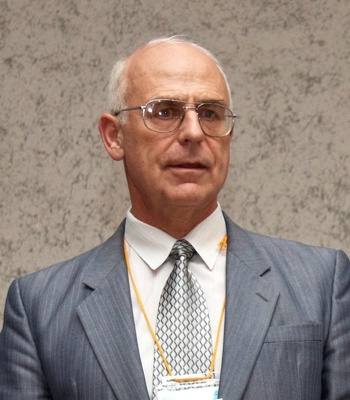Nonlocal problems and their application in mathematical physics and mathematical medicine
Nonlocal problems and their application in mathematical physics and mathematical medicine

Year 2019

Construction of spherically symmetric stationary solutions of the Vlasov-Poisson system of equations describing the stationary distribution of particles in a gravitational field. Obtaining sufficient conditions for confining high-temperature plasma in a “mirror-trap” fusion reactor.
Investigation of reaction-diffusion waves describing blood clotting and the spread of infection. Construction of a mathematical model of the dynamics of HIV infection and the study of controllability and stabilization of the dynamics of infection.
Investigation of the solvability of initial boundary value problems describing the motion of viscoelastic media.
Study of the questions of solvability, correctness, behavior of solutions for large times and controllability of initial boundary value problems for nonlinear evolutionary equations of odd order in spatial variables, including Kawahara and Zakharov-Kuznetsov equations.
Studying the relationship between the scattering matrix and the Weyl function, the characteristic function of a non-self-adjoint operator, and the spectral shift function.
Construction of the theory of boundary value problems for elliptic functional differential equations with affine transformations of independent variables.
Obtaining integral representations and qualitative properties of solutions for the Dirichlet problem in the half-plane for elliptic equations with non-local potential.
Investigation of Fredholm solvability, construction of topological invariants for a symbol of elliptic operators associated with groups of quantized canonical transformations, including operators on compact manifolds, metaplectic operators in Rn of any dimension, wave equation with boundary conditions on a finite cylinder.
Finding the trace formula for the difference between the functions of a perturbed normal operator and the original normal operator.
Establishing the relationship between multivariance, first integrals and integral invariants of given evolutionary problems with non-potential operators.
Proof of the theorem on the isomorphism generated by a non-degenerate difference operator with variable coefficients in Sobolev spaces. Proof of the solvability of the N.N. Krasovskii problem on the complete damping of non-stationary control systems with after-effect.
Development of effective analytic-numerical methods for solving and researching boundary value problems for elliptic equations and systems of differential equations in domains with geometric singularities, and a quasiconformal mapping of such domains. The construction of formulas for analytic continuation of Lauricella-type hypergeometric functions, the development of analytic-numerical methods for the conformal and harmonic mapping of complex domains, and the solution of boundary-value problems in such domains. Description of the structure of formulas for the volumes of hyperbolic simplices of dimension 4 and 5 through the coordinates of the vertices, as well as obtaining the formula for the volume of a spherical tetrahedron through the coordinates of the vertices.
Simulation of the Gaussian distribution and the mixed Gaussian distribution in the presence of outliers through deep neural networks. Dynamic determination of the parameters of neural networks as solutions of a system of differential equations.
Development of difference schemes for identification problems for partial differential equations, and their computer implementation.

Project goals
• The project is devoted to the development of new functional methods for studying linear and nonlinear differential equations and non-local problems and their application to the study of astrophysics, high-temperature plasma and mathematical medicine.